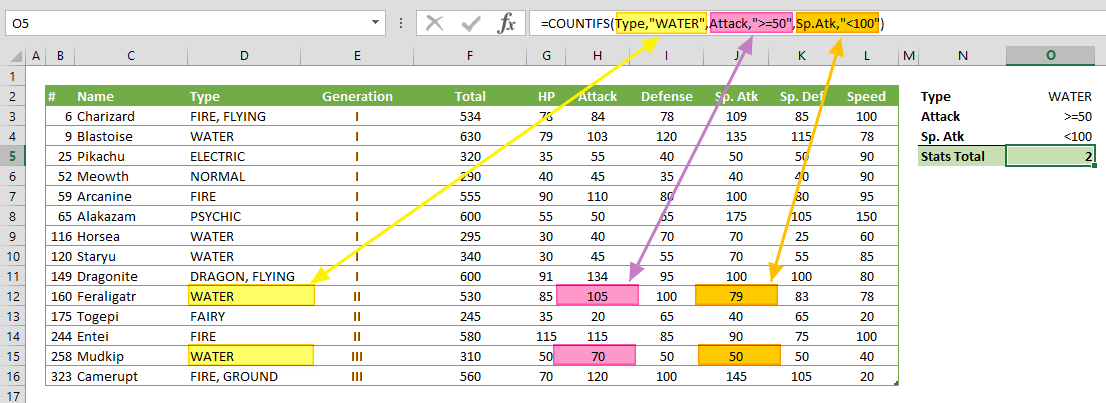COUNTIFS function can count the values in a range of cells, based on some criteria. In this guide, we’re going to show you how to use the COUNTIFS function and cover some examples and error handling methods.

# Supported versions

• (Windows) Excel 2007 and above
• (Mac) Excel for Mac 2011 and above
• Web and mobile versions

# COUNTIFS Syntax

COUNTIFS(criteria_range1, criteria1, [criteria_range2, criteria2], ...)

# Arguments

 criteria_range1 The range of cells that you want to apply the criteria1 against criteria1 The criteria that is applied to criteria_range1 to define which cells to count [criteria_range2, criteria2] Optional. Additional ranges and their associated criteria pairs. You can enter up to 127 range/criteria pairs.

# Examples

Here, we used named ranges to make the formulas easier to read. This is not required.

## Example 1

=COUNTIFS(Total,Type,"WATER",Attack,">=50",Sp.Atk,"<100")
formula counts cells where Type is equal to "WATER", Attack values are equal or greater than 50 and Sp. Atk values are less than 100.Note: SUMIFS function is not case-sensitive. Therefore, "WATER" and "water" criteria will work the same way.

## Example 2

=COUNTIFS(HP,Type,"*FIRE*",Generation,"<>I")
formula counts cells where Type includes "FIRE" string and Generation is not equal to "I". If we use "FIRE" only without asterisks, the COUNTIFS function will skip the "FIRE, GROUND" value.# Tips

• Use the same number of rows and columns for criteria range arguments.
• Good Example: =SUMIFS(G2:G11,F2:F11,">2014",J2:J11,"IT")
• Comparison operators:
 Operator Description Criteria Sample Criteria Meaning = Equal to “=10000” Equal to 10000 <> Not equal to “<>10000” Not equal to 10000 > Greater than “>10000” Greater than 10000 < Less than “>10000” Less than 10000 >= Greater than or equal to “>=10000” Greater than or equal to 10000 <= Less than or equal to “<=10000” Less than or equal to 10000 ? Takes the place of a single character “Admin?” 6-character word starts by “Admin” * Can take the place of any number of characters. “Admin*” Any number of character word starts with “Admin” ~ Use tilde in front of a  question mark or an asterisk to actually find them “Admin~*” Equal to "Admin*"

Note: Wildcards cannot be used for numeric values. Searching a wildcard in a range of numeric values returns no matches.

# Issues

## #VALUE!

The COUNTIFS function returns incorrect results when you use it to match strings longer than 255 characters, or the string #VALUE!.

## Empty Cell Reference

If criteria argument refers an empty cell, the COUNTIFS function evaluates the cell value as 0.